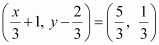# Relations and Functions Chapter 2 Class 11 Maths NCERT PDF

NCERT Solutions for Class 11 Maths Chapter 2′ PDF Quick download link is given at the bottom of this article. You can see the PDF demo, size of the PDF, page numbers, and direct download Free PDF of ‘Ncert Class 11 Maths Chapter 2 Exercise Solution’ using the download button.

### Chapter 2: Relations and Functions

#### 2.1 Overview

This chapter deals with linking a pair of elements from two sets and then introduce relations between the two elements in the pair.

Practically every day of our lives, we pair the members of two sets of numbers.

For example, each hour of the day is paired with the local temperature reading by T.V. Station’s weatherman, a teacher often pairs each set of scores with the number of students receiving that score to see more clearly how well the class has understood the lesson.

Finally, we shall learn about special relations called functions.

#### 2.1.2 Relations

A Relation R from a non-empty set A to a non-empty set B is a subset of the Cartesian product set A × B. The subset is derived by describing a relationship between the first element and the second element of the ordered pairs in A × B

### NCERT Solutions Class 11 Maths Chapter 2 Relations and Functions

1. If, find the values of x and y.

Solution:

Given,As the ordered pairs are equal, the corresponding elements should also be equal.

Thus,

x/3 + 1 = 5/3 and y – 2/3 = 1/3

Solving, we get

x + 3 = 5 and 3y – 2 = 1 [Taking L.C.M and adding]

x = 2 and 3y = 3

Therefore,

x = 2 and y = 1

2. If the set A has 3 elements and the set B = {3, 4, 5}, then find the number of elements in (A × B)?

Solution:

Given, set A has 3 elements and the elements of set B are {3, 4, and 5}.

So, the number of elements in set B = 3

Then, the number of elements in (A × B) = (Number of elements in A) × (Number of elements in B)

= 3 × 3 = 9

Therefore, the number of elements in (A × B) will be 9.

3. If G = {7, 8} and H = {5, 4, 2}, find G × H and H × G.

Solution:

Given, G = {7, 8} and H = {5, 4, 2}

We know that,

The Cartesian product of two non-empty sets P and Q is given as

P × Q = {(pq): ∈ P, q ∈ Q}

So,

G × H = {(7, 5), (7, 4), (7, 2), (8, 5), (8, 4), (8, 2)}

H × G = {(5, 7), (5, 8), (4, 7), (4, 8), (2, 7), (2, 8)}

4. State whether each of the following statements is true or false. If the statement is false, rewrite the given statement correctly.

(i) If P = {mn} and Q = {nm}, then P × Q = {(mn), (nm)}.

(ii) If A and B are non-empty sets, then A × B is a non-empty set of ordered pairs (xy) such that x ∈ A and y ∈ B.

(iii) If A = {1, 2}, B = {3, 4}, then A × (B ∩ Φ) = Φ.

Solution:

(i) The statement is False. The correct statement is:

If P = {mn} and Q = {nm}, then

P × Q = {(mm), (mn), (n, m), (nn)}

(ii) True

(iii) True

5. If A = {–1, 1}, find A × A × A.

Solution:

The A × A × A for a non-empty set A is given by

A × A × A = {(abc): ab∈ A}

Here, It is given A = {–1, 1}

So,

A × A × A = {(–1, –1, –1), (–1, –1, 1), (–1, 1, –1), (–1, 1, 1), (1, –1, –1), (1, –1, 1), (1, 1, –1), (1, 1, 1)}